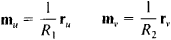Rodrigues Formulas

The following article is from The Great Soviet Encyclopedia (1979). It might be outdated or ideologically biased.

Rodrigues Formulas

expressions for the derivatives of the unit vector m normal to a surface when the net of parametric curves on the surface consists of lines of curvature. If r is the radius vector for the point m on the surface and r1 and R2 are the principal radii of curvature at M, then the Rodrigues formulas can be written aswhere u and ν are parameters along the lines of curvature. These formulas were derived by Rodrigues in 1815.